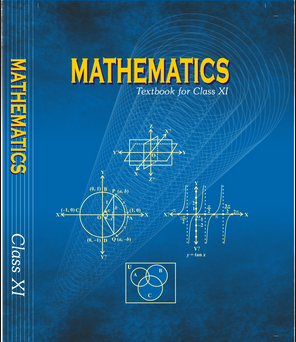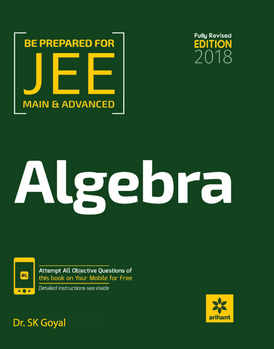# Complex numbers and quadratic equations   Share

## What is Complex numbers and quadratic equations

The complex number and Quadratic Equations:

In Mathematics, one of the most interesting and important topics is Complex Number and Quadratic Equations. Every year you will get at least 1 - 3 questions in JEE Main and other exams, directly and indirectly, the concept of this chapter will be involved in many other chapters, like functions and coordinate geometry. Complex Numbers and Quadratic equations are one of the most important and basic chapters in the preparation of competitive entrance exams. Some students find complex number little challenging to understand and solve the problems initially. But as you solve more and more problems on the Complex number, you will get familiar with this topic. Afterward, the questions will appear easy for you. You will find Quadratic part little easy compared to complex part, the concept of Quadratic part is very straight forward and logically easy to comprehend and correlate.

## Why Complex number and Quadratic Equations:

Complex numbers and quadratic equations both find wide range of application in real-life problem, for example in physics when we deal with circuit and if circuit is involved with capacitor and inductance then we use complex numbers to find the impedance of the circuit and for doing so we use complex numbers to represent the quantities of capacitor and inductance responsible in contribution of impedance.

Quadratic equations are basically parabolic so they are used in throwing a ball (studying the trajectory of ball as equation of trajectory is parabolic), hitting a golf ball, the angle at which we hit golf ball decide the trajectory(parabolic path) of golf ball and hence the distance covered, in rocket propulsion also quadratic equations are used in the calculation of the trajectory of propulsion.

Prepare Online for JEE Main/NEET

Crack JEE 2021 with JEE/NEET Online Preparation Program## After studying this chapter :

1. You will be able to solve the parabolic equations

2. It will be helping you to solve the problem of physics of laws of motion and many motion related chapter

3. The complex part of this chapter will be helping you in electromagnetism and alternating current chapter.

4. It will help you in functions and coordinate geometry and many other mathematics chapters.

5. And obviously, the chapter itself will help you to score some marks in the exam as it gets about 7% weight in jee main and around similar weight in other exams.

## Important topics :

1. Algebra of complex numbers

2. The complex conjugate of complex numbers and properties of complex numbers

3. Modulus and argument of complex numbers

4. Polar form representation and Euler form of complex numbers

5. Finding the square root of complex numbers and complex equations

6. De-moivre’s theorem, cube root and nth  root of unity

7. Vector representation and rotation of complex numbers

8. The discriminant of quadratic equations

9. Nature of roots (in quadratic equations), the relation of coefficient and roots

10. Transformation of quadratic equations and condition of common roots

## Overview of the Complex Numbers and Quadratic Equations:

Complex number: A complex number is the sum of a real number and an imaginary number. For example, 5 + 2i is a complex number. Where 5 is the real part and 2 is the imaginary part.

Algebra : algebra of complex numbers deals with addition, subtraction, multiplication and division of complex numbers, for example addition of 3 + 4i and 9 + 8i is (3+4i) + (9+4i) = 12 + 12i.

Conjugate of complex numbers: the basic thing about conjugate is that they differ by the sign of imaginary part, if the imaginary part of the number is +ve then it’s conjugate imaginary part becomes -ve.

For example

z = 2 + 2i

z= 2 - 2iModulus and argument of a complex number: modulus is of a complex number is basically the distance of the point from the origin, so if z = a+bi is the complex number then it |z| = .  The argument is basically the angle complex number z makes with the +ve x-axis.

Quadratic equations: If the degree of a polynomial equation is 2 then it is said to be the quadratic equation. Value of x for which quadratic equations is satisfied is called its roots.

The standard form of a quadratic equation is ax2 + b x + c = 0, Where a, b and c are constant and is called the coefficient of the equation.

The discriminant of quadratic equations: Discriminant of quadratic equations is represented by D = b2 - 4ac. The discriminant of a quadratic equation is used in the Sridharacharya formula of quadratic equations to find the roost which is

Putting + and - one after another we get two required solutions of the equation, if D>0 then both roots are different if D = 0 then roots are same and if D<0 then the roots are complex and they exist in pairs of conjugate numbers.

Relation of coefficient and roots:If       are the two roots then the sum of roots are related to coefficient as   and product of roots is related with coefficient as  .

## How to prepare Complex Numbers and Quadratic Equations:

Complex numbers and quadratic equations are a topics topic for functions and for some concept in coordinate geometry, so you must be through with this chapter.

1. Start with understanding basic concepts like Definition of Complex Number, Integral Powers of iota (), Representation of a Complex number in various forms.

2. Then go to the algebra of complex numbers, Argand plane, modulus and argument of complex number and triangle important is also an important concept.

3. After studying these concepts go through solved examples and then go to mcq and practice the problem to make sure you understood the topic.

4. Solve the questions of the books which you are following and then go to previous year papers.

5. For the quadratic equation, you should do the same things in the same order as mentioned above for complex numbers.

6. While going through concept make sure you understand the derivation of formulas and try to derive them by your own, as many times you will not need the exact formula but some steps of derivation will be very helpful to solve the problem if you understand the derivation it will boost your speed in problem-solving.

7. Since both topics are geometrically involved try to understand and relate the things with geometry and graph as the graph makes visualization of the problem easy and hence it makes the problem easy for us to solve.

8. At the end of chapter try to make your own short notes for quick revision, make a list of formula to revise quickly before exams or anytime when you required to revise the chapter, it will save lots of time for you.

## Best Books for the Preparation of This Chapter:

First, finish all the concept, example and questions given in NCERT Maths Book. You must be thorough with the theory of NCERT. Then you can refer to the book Algebra Arihant by Dr. SK goyal or RD Sharma or Cengage Mathematics Algebra but make sure you follow any one of these not all. Complex numbers and quadratic equations are explained very well in these books and there are an ample amount of questions with crystal clear concepts. Choice of reference book depends on person to person, find the book that best suits you the best, depending on how well you are clear with the concepts and the difficulty of the questions you require.

## Maths Chapter-wise Notes for Engineering exams

 Chapters Chapters Name Chapter 1 Sets, Relations, and Functions Chapter 3 Matrices and Determinants Chapter 4 Permutations and Combinations Chapter 5 Binomial Theorem and its Simple Applications Chapter 6 Sequence and Series Chapter 7 Limit, Continuity, and Differentiability Chapter 8 Integral Calculus Chapter 9 Differential Equations Chapter 10 Coordinate Geometry Chapter 11 Three Dimensional Geometry Chapter 12 Vector Algebra Chapter 13 Statistics and Probability Chapter 14 Trigonometry Chapter 15 Mathematical Reasoning Chapter 16 Mathematical Induction

### Topics from Complex numbers and quadratic equations

• Representation of complex numbers in the form of a+ib and their represenation in a plane ( JEE Main, SRMJEEE, KVPY SA, KVPY SB/SX, KEAM ) (2 concepts)
• Complex numbers as ordered pairs of reals ( JEE Main, SRMJEEE, KVPY SA, KVPY SB/SX, KEAM ) (6 concepts)
• Algebra of complex numbers ( JEE Main, SRMJEEE, KVPY SA, KVPY SB/SX, KEAM ) (10 concepts)
• Modulus and argument(or amplitude) of a complex number ( JEE Main, SRMJEEE, KVPY SA, KVPY SB/SX, KEAM ) (50 concepts)
• Triangle inequality ( JEE Main, SRMJEEE, KVPY SA, KVPY SB/SX, KEAM ) (4 concepts)
• Square root of a complex number ( JEE Main, SRMJEEE, KVPY SA, KVPY SB/SX, KEAM ) (2 concepts)
• Quadratic equations in real and complex number system and their solutions ( JEE Main, SRMJEEE, KVPY SA, KVPY SB/SX, KEAM ) (10 concepts)
• Relation between roots and co-efficients, nature of roots, formation of quadratic equations with given roots ( JEE Main, SRMJEEE, KVPY SA, KVPY SB/SX, KEAM ) (76 concepts)
• Argand diagram ( JEE Main, SRMJEEE, KVPY SA, KVPY SB/SX, KEAM ) (16 concepts)
• Complex Number ( JEE Main, SRMJEEE, KVPY SA, KVPY SB/SX, KEAM ) (10 concepts)
• Geometrical Representation and Different Forms of Complex Number ( JEE Main, SRMJEEE, KVPY SA, KVPY SB/SX, KEAM ) (14 concepts)
• De-Moivre's Theorem ( JEE Main, SRMJEEE, KVPY SA, KVPY SB/SX, KEAM ) (2 concepts)
• Cube Root and nth Root of Unity ( JEE Main, SRMJEEE, KVPY SA, KVPY SB/SX, KEAM ) (4 concepts)
• Geometry with Complex Number ( JEE Main, SRMJEEE, KVPY SA, KVPY SB/SX, KEAM ) (6 concepts)
• Quadratic Equations and Roots ( JEE Main, SRMJEEE, KVPY SA, KVPY SB/SX, KEAM ) (6 concepts)
• Transformation of Quadratic Equations and Common Roots ( JEE Main, SRMJEEE, KVPY SA, KVPY SB/SX, KEAM ) (4 concepts)
• Graphs of Quadratic Equations ( JEE Main, SRMJEEE, KVPY SA, KVPY SB/SX, KEAM ) (8 concepts)
• Quadratic Expression in two Variables ( JEE Main, SRMJEEE, KVPY SA, KVPY SB/SX, KEAM ) (2 concepts)
• Location of Roots ( JEE Main, SRMJEEE, KVPY SA, KVPY SB/SX, KEAM ) (6 concepts)
• Polynomial Equation and Remainder Theorem ( JEE Main, SRMJEEE, KVPY SA, KVPY SB/SX, KEAM ) (2 concepts)
• Other Types of Equations and their Inequalities ( JEE Main, SRMJEEE, KVPY SA, KVPY SB/SX, KEAM ) (8 concepts)

### Important Books for Complex numbers and quadratic equations

••Exams
Articles
Questions